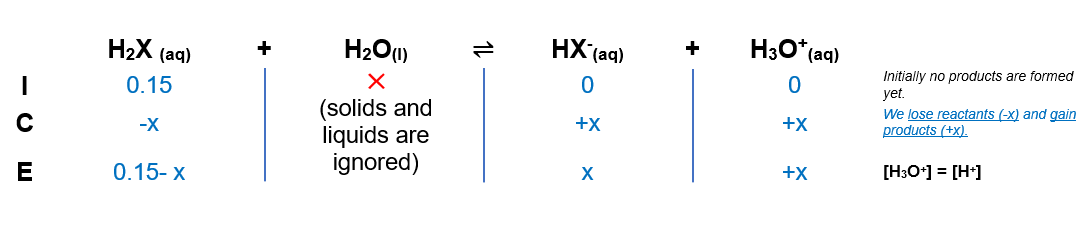# Problem: What is the concentration of X2- in a 0.150 M solution of the diprotic acid H2X? For H2X, Ka1  = 4.5 x 10 - 6 and Ka2  = 1.2 x 10 - 11.

###### FREE Expert Solution

(1) Setup an ICE Chart(2) Calculate for HX- and H3O+

$\overline{){{\mathbf{K}}}_{{\mathbf{a}}}{\mathbf{=}}\frac{\mathbf{products}}{\mathbf{reactants}}{\mathbf{=}}\frac{\mathbf{\left[}{\mathbf{H}}_{\mathbf{3}}{\mathbf{O}}^{\mathbf{+}}\mathbf{\right]}\mathbf{\left[}{\mathbf{C}}_{\mathbf{2}}{\mathbf{H}}_{\mathbf{5}}{{\mathbf{CO}}_{\mathbf{2}}}^{\mathbf{-}}\mathbf{\right]}}{\mathbf{\left[}{\mathbf{C}}_{\mathbf{2}}{\mathbf{H}}_{\mathbf{5}}{\mathbf{CO}}_{\mathbf{2}}\mathbf{H}\mathbf{\right]}}}$

97% (223 ratings)###### Problem Details

What is the concentration of X2- in a 0.150 M solution of the diprotic acid H2X? For H2X, Ka1  = 4.5 x 10 - 6 and Ka2  = 1.2 x 10 - 11.

Frequently Asked Questions

What scientific concept do you need to know in order to solve this problem?

Our tutors have indicated that to solve this problem you will need to apply the Diprotic Acid concept. You can view video lessons to learn Diprotic Acid. Or if you need more Diprotic Acid practice, you can also practice Diprotic Acid practice problems.

What professor is this problem relevant for?

Based on our data, we think this problem is relevant for Professor Davis' class at UCF.